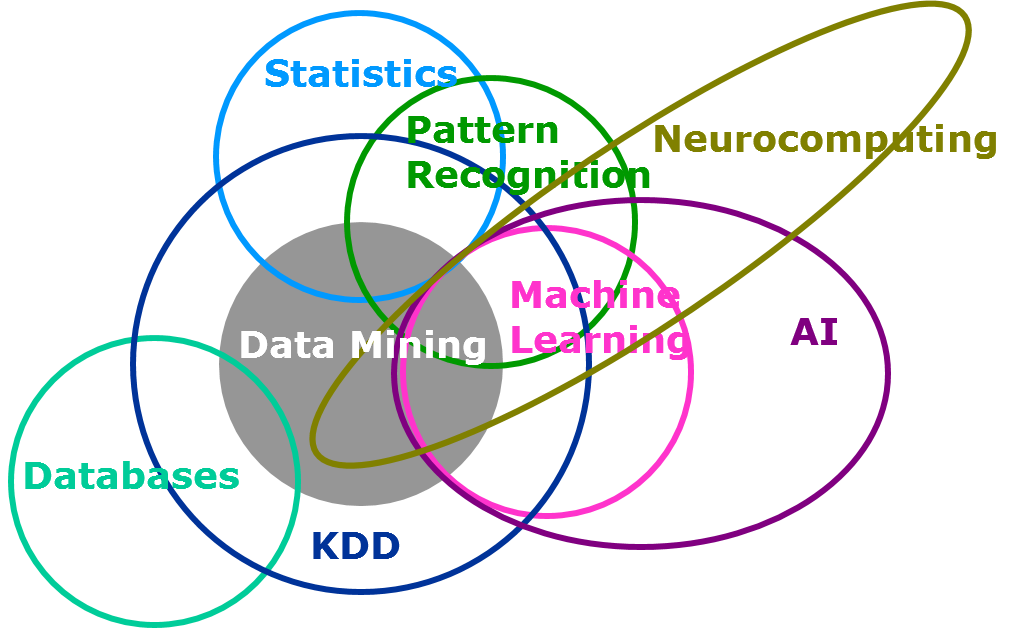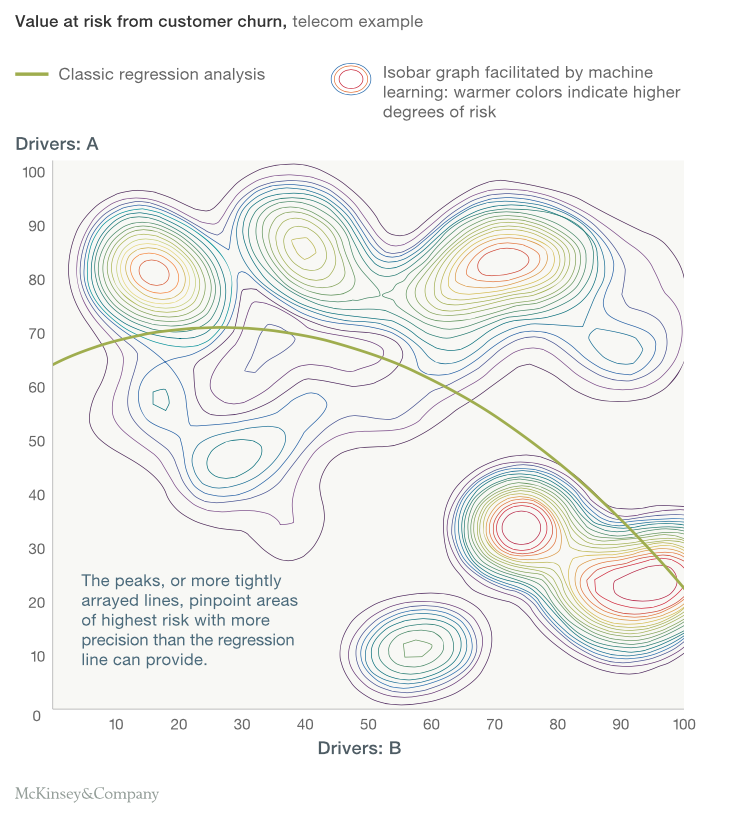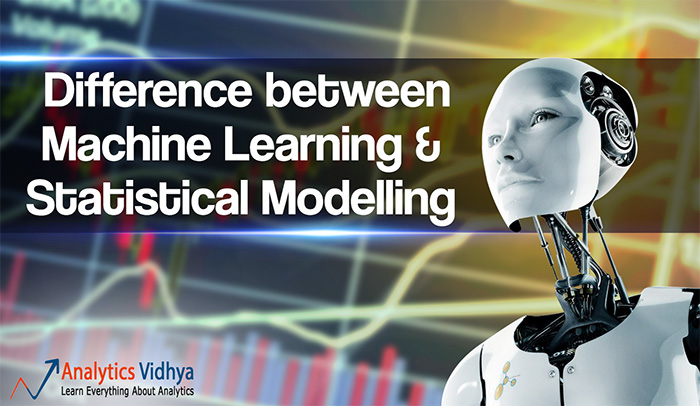Home » Difference between Machine Learning & Statistical Modeling

# Difference between Machine Learning & Statistical Modeling

One of the most common question, which gets asked at various data science forums is:

What is the difference between Machine Learning and Statistical modeling?

I have been doing research for the past 2 years. Generally, it takes me not more than a day to get clear answer to the topic I am researching for. However, this was definitely one of the harder nuts to crack. When I came across this question at first, I found almost no clear answer which can layout how machine learning is different from statistical modeling. Given the similarity in terms of the objective both try to solve for, the only difference lies in the volume of data involved and human involvement for building a model. Here is an interesting Venn diagram on the coverage of machine learning and statistical modeling in the universe of data science (Reference: SAS institute)In this article, I will try to bring out the difference between the two to the best of my understanding. I encourage more seasoned folks of this industry to add on to this article, to bring out the difference.

Before we start, let’s understand the objective behind what we are trying to solve for using either of these tools. The common objective behind using either of the tools is Learning from Data. Both these approaches aim to learn about the underlying phenomena by using data generated in the process.

Now that it is clear that the objective behind either of the approaches is same, let us go through their definition and differences.

Before you proceed: Machine learning basics for a newbie

## Definition:

Machine Learning is

an algorithm that can learn from data without relying on rules-based programming.

Statistical Modelling is

formalization of relationships between variables in the form of mathematical equations.

For people like me, who enjoy understanding concepts from practical applications, these definitions don’t help much. So, let’s look at a business case here.

Let us now see an interesting example published by McKinsey differentiating the two algorithms :

Case : Understand the risk level of customers churn over a period of time for a Telecom company

Data Available : Two Drivers – A & B

What McKinsey shows next is an absolute delight! Just stare at the below graph to understand the difference between a statistical model and a Machine Learning algorithm.What did you observe from the above graph?  Statistical model is all about getting a simple formulation of a frontier in a classification model problem. Here we see a non linear boundary which to some extent separates risky people from non-risky people. But when we see the contours generated by Machine Learning algorithm, we witness that statistical modeling is no way comparable for the problem in hand to the Machine Learning algorithm. The contours of machine learning seems to capture all patterns beyond any boundaries of linearity or even continuity of the boundaries. This is what Machine Learning can do for you.

If this is not an inspiration enough, machine learning algorithm is used in recommendation engines of YouTube / Google etc. which can churn trillions of observations in a second to come up with almost a perfect recommendation. Even with a laptop of 16 GB RAM I daily work on datasets of millions of rows with thousands of parameter and build an entire model in not more than 30 minutes. A statistical model on another hand needs a supercomputer to run a million observation with thousand parameters.## Differences between Machine Learning and Statistical Modeling:

Given the flavor of difference in output of these two approaches, let us understand the difference in the two paradigms, even though both do almost similar job :

1. Schools they come from
2. When did they come into existence?
3. Assumptions they work on
4. Type of data they deal with
5. Nomenclatures of operations and objects
6. Techniques used
7. Predictive power and human efforts involved to implement

All the differences mentioned above do separate the two to some extent, but there is no hard boundary between Machine Learning and statistical modeling.

### They belong to different schools

Machine Learning is

a subfield of computer science and artificial intelligence which deals with building systems that can learn from data, instead of explicitly programmed instructions.

Statistical Modelling is

a subfield of mathematics which deals with finding relationship between variables to predict an outcome

### They came up in different eras

Statistical modeling has been there for centuries now. However, Machine learning is a very recent development. It came into existence in the 1990s as steady advances in digitization and cheap computing power enabled data scientists to stop building finished models and instead train computers to do so. The unmanageable volume and complexity of the big data that the world is now swimming in have increased the potential of machine learning—and the need for it.

### Extent of assumptions involved

Statistical modeling work on  a number of assumption. For instance a linear regression assumes :

1. Linear relation between independent and dependent variable
2. Homoscedasticity
3. Mean of error at zero for every dependent value
4. Independence of observations
5. Error should be normally distributed for each value of dependent variable

Similarly Logistic regressions comes with its own set of assumptions. Even a non linear model has to comply to a continuous segregation boundary. Machine Learning algorithms do assume a few of these things but in general are spared from most of these assumptions. The biggest advantage of using a Machine Learning algorithm is that there might not be any continuity of  boundary as shown in the case study above. Also, we need not specify the distribution of dependent or independent variable in a machine learning algorithm.

### Types of data they deal with

Machine Learning algorithms are wide range tools. Online Learning tools predict data on the fly. These tools are capable of learning from trillions of observations one by one. They make prediction and learn simultaneously. Other algorithms like Random Forest and Gradient Boosting are also exceptionally fast with big data. Machine learning does really well with wide (high number of attributes) and deep (high number of observations). However statistical modeling are generally applied for smaller data with less attributes or they end up over fitting.

### Naming Convention

Here are a names which refer to almost the same things :### Formulation

Even when the end goal for both machine learning and statistical modeling is same, the formulation of two are significantly different.

In a statistical model, we basically try to estimate the function f in

`Dependent Variable ( Y )  = f(Independent Variable) + error function`

Machine Learning takes away the deterministic function “f” out of the equation. It simply becomes

`Output(Y)  ----- >  Input (X)`

It will try to find pockets of X in n dimensions (where n is the number of attributes), where occurrence of Y is significantly different.

### Predictive Power and Human Effort

Nature does not assume anything before forcing an event to occur.

So the lesser assumptions in a predictive model, higher will be the predictive power. Machine Learning as the name suggest needs minimal human effort. Machine learning works on iterations where computer tries to find out patterns hidden in data. Because machine does this work on comprehensive data and is independent of all the assumption, predictive power is generally very strong for these models. Statistical model are mathematics intensive and based on coefficient estimation. It requires the modeler to understand the relation between variable before putting it in.

### End Notes

However, it may seem that machine learning and statistical modeling are two different branches of predictive modeling, they are almost the same. The difference between these two have gone down significantly over past decade. Both the branches have learned from each other a lot and will further come closer in future. I hope we motivated you enough to acquire skills in each of these two domains and then compare how do they compliment each other.

If you are interested in picking up machine learning algorithms, we have just the right thing coming up for you. We are in process of building a learning path for machine learning which will be published soon.

Let us know what you think is the difference between machine learning and statistical modeling? Do you have any case study to point out the differences between the two?

### If you like what you just read & want to continue your analytics learning, subscribe to our emails, follow us on twitter or like our facebook page.

You can also read this article on our Mobile APP•manizoya says:

Brilliant article Tavish and beautifully explained. This fact has bothered me far too long and I have formed my own raw interpretations. Statistics and models derived from statistics primarily were concerned with numbers and numerical outputs. This probably was one more reason for machine learning to step in and supply the algorithms to run decision trees, support vector machines etc which work well on categorical data. Also historically the biggest application of statistics has been in hypothesis testing – to prove or disprove something based on numbers. Prediction was never really the most important goal of statisticians, which is where again machine learning comes in.

•SRK says:

Thanks Tavish for the excellent post with examples.

•Ks Loke says:

statistics require assumption on the distribution of data, Machine Learning may or may not. Machine learning can be employed in other areas, e.g. can learn sequence of actions or tasks (play games, drive car,etc).

•Jayant says:

Well, statistical learning and machine learning are more or less same. You are assuming that all statistical techniques are parametric but its not so. The disciplines evolved independently but they take more or less same path and have different nomenclature. The difference between the two ends here. Machine Learning techniques such as penalized regression are very much a result from statistical branch. I can confidently say Machine Learning was going on much before 1990. It was just known more popularly as Artificial Intelligence. And what about Gaussian kernel in a Neural Network? Assumption used in Neural Network activation. Who will you credit this? Mathematicians or Computer Scientists or both?

•Doug Dame says:

Nice write-up.

One additional difference worth mentioning between machine learning and traditional statistical learning is the philosophical approach to model building. Traditional statistical learning almost always assumes there is one underlying “data generating model”, and good practice requires that the analyst build a model using inputs that have a logical basis for being somehow related to the independent variable. You quantify what you suspect, so to speak. Throwing tons of inputs into the prospective model, just because you have them available, is considered fishing for an answer and is frowned upon.

In contrast, machine learning requires essentially no a priori beliefs about the nature of the true underlying relationships, and doesn’t even necessary expect that there is just one best model waiting to be discovered. Throw in all the data you can find, and let the machine empirically discover whatever relationships there are in the data, be they obvious and already known main effects, or some fifth-order interaction never previously described in the literature.

Also, because many machine learning algorithms are capable of extremely flexible models, and often start with a large set of inputs that has not been reviewed item-by-item on a logical basis, the risk of overfitting or finding spurious correlations is usually considerably higher than is the case for most traditional statistical models. This concern has driven much of the work on cross-validation and various kinds of penalized models in the past 25-30 years.

•Tavish says:

Thanks for the input Doug.

•John Thompson says:

Brilliant article. I will amplify via social media. I have a question, in your Venn diagram Statistics and ML only share a small boundary, but in the text you argue that they are the same. Do you need another circle that is Statistical Modelling?

•Tavish says:

Hi John,
You are right, the circle for statistical modelling needs to overlayed on top of the Venn diagram. However, this diagram was just for illustration purpose that all these domains have a big overlap.

Tavish

•sandhyac23 says:

Very informative. Thank You. However, I need suggestions to learn machine learning.I have been going through coursera.org to learn machine learning. But need to learn something that is practically used at work. As of now, I can work on statistical models using logistic regression in SAS. Please suggest.

•JoaoVictor says:

I guess it was an unfair comparison.

Statistical modelling is a narrow field from a big science.
It’s not a matter of words but I’d compare Machine Learning to Statistical Learning.
That would’ve been better, in my opinion.

When you read top kaggler’s teachings they usually give a great amount of attention to assumptions.
Owen Zhang, kaggle #1, says “think more, try less”.
But what some people usually do is running a lot of famous algorithms and try squeezing something good from there.

I wouldn’t tell my students “So the lesser assumptions in a predictive model, higher will be the predictive power.” because that is not a truth neither the opposite is.
I guess the statistical assumptions acquired a new name in ML (maybe priori beliefs) and the way researchers deal with them changed but applying a data driven approach without knowing the data’s and algorithm’s nature may lead someone to a spurious result.

Keep rocking, Tavish!
Success!

•Tavish says:

Hi Joao,
You made some very valid points. You definetely need to validate assumptions to be a rockstar in the world of Machine Learning. I think you took this point the wrong way. I was trying to differentiate two very close school of thoughts : Machine Learning and Statistical Modelling. While comparing the two, ML assumes a lot less compared to Statistical model (however there is no solid line seperating the two). But while building models to compete on Kaggle you need to mix the understanding of data and power of machine learning. Hope this gives a better perspective.
Tavish

•Uyanga Sumiya says:

Great article explaining the difference. Thank you. It’s so helpful.

•jacob says:

Excellent article. If yo draw an analogy to stock market investing, it is like Fundamental v/s Technical Analysis (Fundamental relates more to Statistical technique and Technical Analysis to Machine learning)

•jacob says:

Excellent article. If you draw an analogy to stock market investing, it is like Fundamental v/s Technical Analysis (Fundamental relates more to Statistical technique and Technical Analysis to Machine learning)

•Mbaye Babacar Gueye says:

Excellent article. The Machine learning is very suitable for inverse (ill-posed) problem ( Hadamard) where we know that there is a relationship between inputs and outputs but we can’t state it. I discuss this in my article “Neural approach to inverting complex system: Application to ocean salinity profile estimation from surface parameters” (link http://www.sciencedirect.com/science/article/pii/S0098300414001782).
Thx

•Parimoj Malhotra says:

Very nice article. Its really helpful.

•Sushanta Chakraborty says:

Short, sweet and useful article. Thanks.
The Venn Diagram placement was very effective, although not every topic there is about predictions. Still, makes sense.

•Vishal says:

There was no mention of the type of data – structured/semi structured/unstructured and which methods do just to either of these type of data.

•Vijay Gupta says:

The basic difference is that Machine Learning is derived from a BayesIan approach, from Bayes Ian Learning. It requires assumptions on the priors. It is called “Learning” because the machine (a set of matrices and equations) updates/learns the prior and posterior as new data arrives. The basic difference is the difference between Frequentist/parametric and BayesIan. Your article is a bit misguiding.

•Ganesh says:

Article is good but not explaining much deepen difference. But, whatever Vijay said that is correct in addition Vijay one point i want to make that not every algorithm in machine learning assumes distributional assumption while in Bayesian we find posterior distribution which is again like making an assumption.
Need to find more on the difference.

•jatin pal singh says:

A good article well directed examples

•Sudhanva says:

Good Article….

•Phanikumar says:

Thanks for the enlightenment. Brilliant article

•zahra oveysi says:

if you study statistics you would know data better than others.
so you can determine which kind of analysis is better when its necessary
finally i would like to recommend statistics to all people who want to be pro data miners.
in despite of big diffrences between them they have the same goal…prediction…
so i will promise the combination of them could be much usefull.